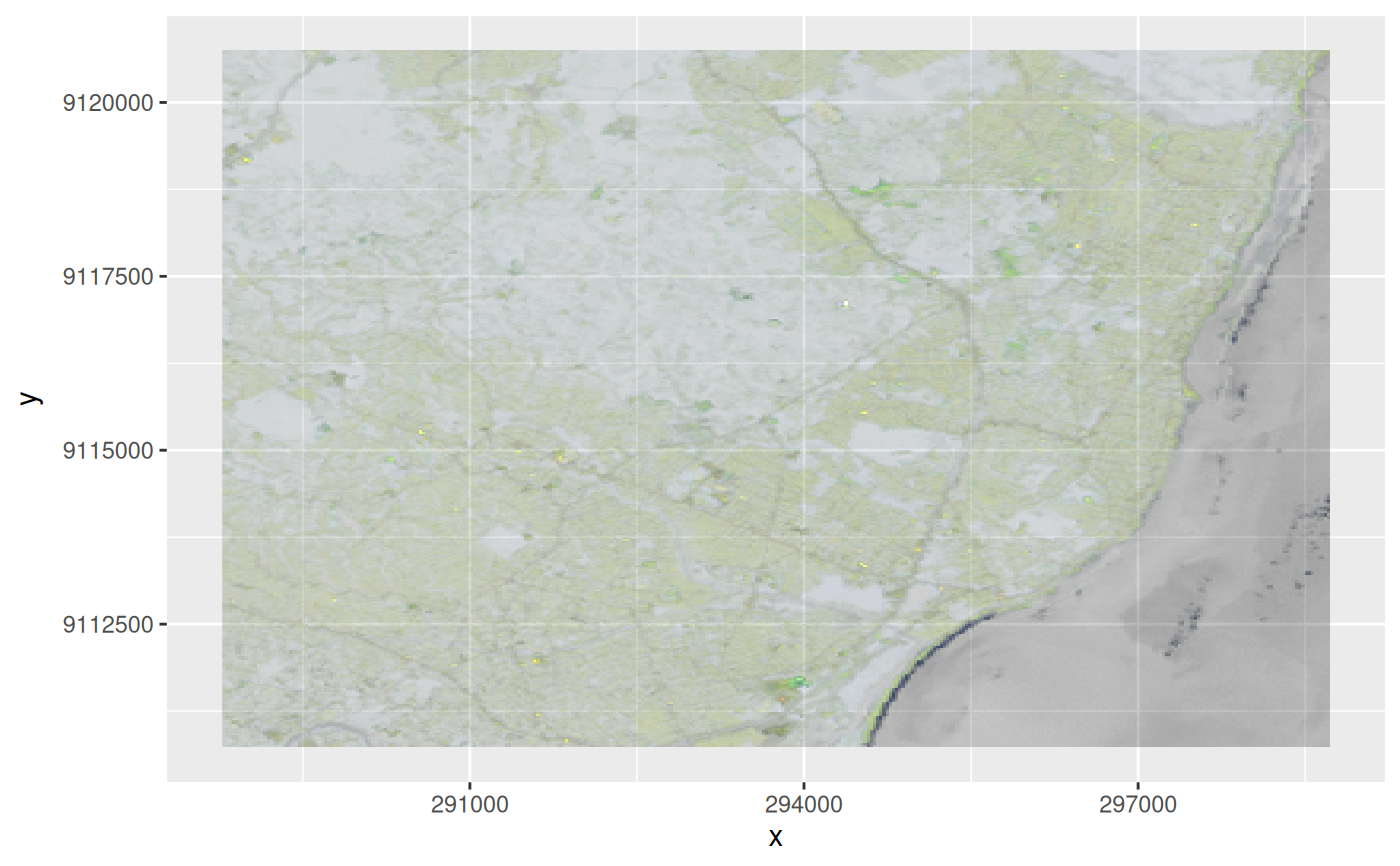reduce dimension to rgb (alpha) hex values

st_rgb(
x,
dimension = 3,
use_alpha = dim(x)[dimension] == 4,
maxColorValue = 255L,
probs = c(0, 1),
stretch = NULL
)

## Arguments

x

object of class stars

dimension

dimension name or number to reduce

use_alpha

logical; if TRUE, the fourth band will be used as alpha values

maxColorValue

integer; maximum value for colors

probs

probability values for quantiles used for stretching by "percent".

stretch

logical or character; if TRUE or "percent", each band is stretched to 0 ... maxColorValue by "percent clip" method using probs values. If "histogram", a "histogram equalization" is performed (probs values are ignored). If stretch is NULL or FALSE, no stretching is performed. Other character values are interpreted as "percent" and a message will be printed.

## Details

the dimension's bands are mapped to red, green, blue, alpha; if a different ordering is wanted, use [.stars to reorder a dimension, see examples

## Examples

tif = system.file("tif/L7_ETMs.tif", package = "stars")
st_rgb(x[,,,3:1])
#> stars object with 2 dimensions and 1 attribute
#> attribute(s):
#>  L7_ETMs.tif
#>  Length:122848
#>  Class :character
#>  Mode  :character
#> dimension(s):
#>   from  to  offset delta                     refsys point values x/y
#> x    1 349  288776  28.5 SIRGAS 2000 / UTM zone 25S FALSE   NULL [x]
#> y    1 352 9120761 -28.5 SIRGAS 2000 / UTM zone 25S FALSE   NULL [y]
r = st_rgb(x[,,,c(6,5,4,3)], 3, use_alpha=TRUE) # now R=6,G=5,B=4,alpha=3
if (require(ggplot2)) {
ggplot() + geom_stars(data = r) + scale_fill_identity()
}r = st_rgb(x[,,,3:1],
probs = c(0.01, 0.99),
stretch = "percent")
plot(r)
#> Error in image.stars(x, ..., axes = axes, breaks = breaks, col = col,     key.pos = key.pos, key.width = key.width, key.length = key.length,     main = NULL): rgb plotting not supported on this device
r = st_rgb(x[,,,3:1],
probs = c(0.01, 0.99),
stretch = "histogram")
plot(r)
#> Error in image.stars(x, ..., axes = axes, breaks = breaks, col = col,     key.pos = key.pos, key.width = key.width, key.length = key.length,     main = NULL): rgb plotting not supported on this device